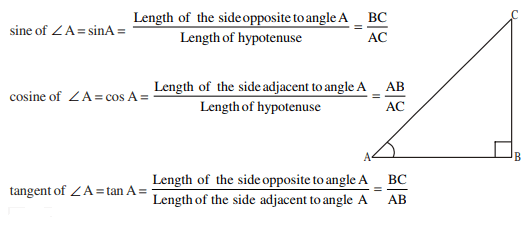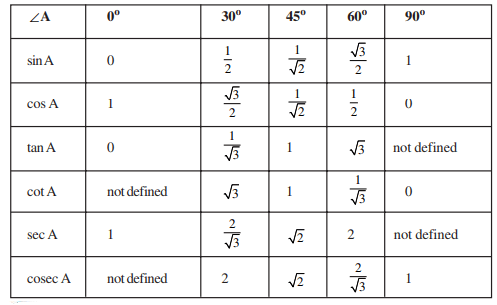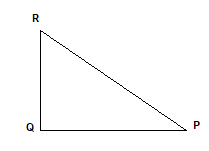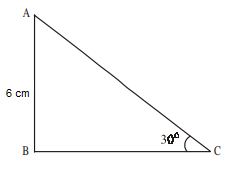# AP SSC Class 10 Maths Chapter 11 Trigonometry

Trigonometry is a branch of Mathematics that deals with the study of relationships between the sides and angles of triangles. In AP Class 10 Maths Chapter 11 Trigonometry, we learn about various properties of a right-angled triangle and trigonometric ratios of angles based on sides only.

Check out these AP Class 10 Maths Chapter 11 Trigonometry solutions and notes will help the students to understand the concepts and master the subject for the board exams.

## Trigonometric Ratios

The ratios of the sides of a right triangle are known as trigonometric ratios. The three common trigonometric ratios are sine (sin), cosine (cos), and tangent (tan).

• The ratio of the opposite side of an angle and length of the hypotenuse is constant in all similar right angle triangles. This ratio is named as “sine” of that angle.
• The ratio of the adjacent side of an angle and length of the hypotenuse is constant in all similar right triangles. This ratio is known as the “cosine” of that angle.
• The ratio of the opposite side and the adjacent side of an angle is constant and is known as the “tangent” of that angle.

In a right angle triangle, the trigonometric ratios can be defined as follows:The values of the trigonometric ratios of the angles 0°, 30°, 60° and 90° are given in a table below### Class 10 Maths Chapter 11 Trignometry Questions

1. In a right angle triangle PQR, 8 cm, 15 cm and 17 cm are the lengths of PQ, QR and RP, respectively. Then, find out sin A, cos A and tan A.

Solution:

In the right angle PQR, the right angle is at Q.

The diagram of the right angle triangle PQR is given as followsPR = Hypotenuse, PQ = Adajacent Side and QR = Opposite side

$\sin A =\frac{Opposite \,side}{Hypotenuse}$

Substituting the values in the aboove equation, we get

$\sin A =\frac{15}{17}$

$\cos A =\frac{Adjacent}{Opposite}$

Substituting the values, we get

$\cos A =\frac{8}{15}$

$\tan A =\frac{Opposite}{Adjacent}$

Substituting the values, we get

$\tan A =\frac{15}{8}$

1. In ∆ABC, right angle is at B, AB = 6 cm and ∠ACB = 30°. Determine the lengths of the sides BC and AC.Solution:

To find the length of side BC, let us choose the trigonometric ratio involving BC and the given side AB.

Therefore,

$\tan C=\frac{AB}{BC}$

Substituting the values, we get

$\tan 30^{\circ}=\frac{6}{BC}$ $\frac{1}{\sqrt{3}}=\frac{6}{BC}$ $BC=6\sqrt{3}\,cm$

Now using Pythagorus theorem,

$AC^{2}=AB^{2}+BC^{2}$ $AC^{2}=6^{2}+(6\sqrt{3})^{2}$ $AC^{2}=36+108=144$ $AC=\sqrt{144}=12\,cm$

AC = 12 cm

Stay tuned to BYJU’S to get the latest notification on SSC exam along with AP SSC model papers, exam pattern, marking scheme and more.